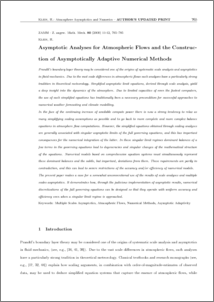Repository: Freie Universität Berlin, Math Department

# Asymptotic Analyses for Atmospheric Flows and the Construction of Asymptotically Adaptive Numerical Methods

Klein, R. (2000) Asymptotic Analyses for Atmospheric Flows and the Construction of Asymptotically Adaptive Numerical Methods. ZAMM ·Zeitschrift für angewandte Mathematik und Mechanik, 80 (11-12). pp. 765-785. ISSN 0044-2267Preview

182kB

## Abstract

Prandtl’s boundary layer theory may be considered one of the origins of systematic scale analysis and asymptotics in fluid mechanics. Due to the vast scale differences in atmospheric flows such analyses have a particularly strong tradition in theoretical meteorology. Simplified asymptotic limit equations,derive d through scale analysis,yield a deep insight into the dynamics of the atmosphere. Due to limited capacities of even the fastest computers, the use of such simplified equations has traditionally been a necessary precondition for successful approaches to numerical weather forecasting and climate modelling. In the face of the continuing increase of available compute power there is now a strong tendency to relax as many simplifying scaling assumptions as possible and to go back to more complete and more complex balance equations in atmosphere flow computations. However,the simplified equations obtained through scaling analyses are generally associated with singular asymptotic limits of the full governing equations,and this has important consequences for the numerical integration of the latter. In these singular limit regimes dominant balances of a few terms in the governing equations lead to degeneracies and singular changes of the mathematical structure of the equations. Numerical models based on comprehensive equation systems must simultaneously represent these dominant balances and the subtle,but important,deviations from them. These requirements are partly in contradiction,and this can lead to severe restrictions of the accuracy and/or efficiency of numerical models. The present paper makes a case for a somewhat unconventional use of the results of scale analyses and multiple scales asymptotics. It demonstrates how,thr ough the judicious implementation of asymptotic results,numeric al discretizations of the full governing equations can be designed so that they operate with uniform accuracy and efficiency even when a singular limit regime is approached.

Item Type: Article Multiple Scales Asymptotics,A tmospheric Flows,Numerical Methods,Asymptotic Adaptivity Mathematical and Computer Sciences > Mathematics > Applied Mathematics Department of Mathematics and Computer Science > Institute of Mathematics > Geophysical Fluid Dynamics Group 480 Ulrike Eickers 26 Jun 2009 08:32 03 Mar 2017 14:40

Repository Staff Only: item control page